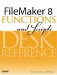# PV()

Category: Financial

 Syntax: PV ( payment; interestRate; periods )

Parameters:

paymentA number (or numeric expression) representing a payment amount made per period.

interestRateA decimal number (or numeric expression) representing the interest rate per period.

periodsThe number of periods of the loan.

Data type returned: Number

Description:

The Present Value formula tells you what money expected in the future is worth today. The PV() function returns the present value of a series of equal payments made at regular intervals, and assumes a fixed interestRate per interval.

Examples:

Imagine you have won \$1,000,000 in a lottery. You have been offered either \$50,000 per year for the next 20 years, or a one-time lump sum payment now of \$700,000. Which option should you choose?

Assuming an inflation rate of 3% per year, the present value of the future payments is determined by

``` PV (50000; .03; 20)
```

which returns 743873.74.

If you assume a 4% inflation rate, the present value of the future payments decreases to 679516.31. So, depending on your assumptions about inflation rates, you may or not be better off taking the lump sum payment.

As another example, consider the question of whether it would be better for someone to give you \$10 today, or \$1 per year for the next 10 years. You can use the PV() function to tell you the present value of receiving \$1 for 10 years. Assuming an inflation rate of 3%, the formula PV (1, .03, 10) shows that the present value of the future income is only \$8.53.FileMaker 8 Functions and Scripts Desk Reference
ISBN: 0789735113
EAN: 2147483647
Year: 2004
Pages: 352